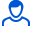Content: teorver_5594.doc (28.00 KB)

Positive responses: 0
Negative responses: 0

Refunds: 05594. x̅=53, n=36, σ=0.5. Based on the sample size n=36, the arithmetic mean x̅в=53 was found. Assuming that X is a normally distributed random variable, find the confidence interval that, with reliability γ=0.999, covers the unknown mathematical expectation a if the general standard deviation σ=0.5
Detailed solution. Decorated in Microsoft Word 2003 (Quest decided to use the formula editor)
No feedback yet